## Mutual Coupling

Mutual coupling is the electromagnetic interaction between the antenna elements in an array. The current developed in each antenna element of an array depends on their own excitation and also on the contributions from adjacent antenna elements. Mutual coupling is inversely proportional to the spacing between the different antenna elements in an array. Mutual coupling in an array causes:

• Changes in the radiation pattern of the array

• Changes in the input impedance of the individual antenna elements in an array

To characterize mutual coupling, you can use mutual impedance, S-parameters, a coupling matrix, or an embedded element pattern.

### Active or Scan Impedance

Active impedance, or scan impedance, is the input impedance of each antenna element in an array, when all elements are excited.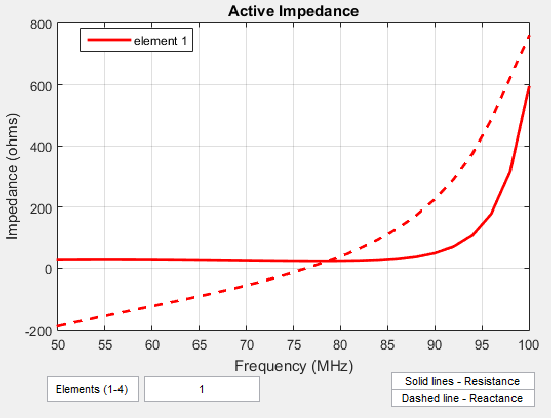The active impedance of an array depends on:

• Array configuration

• Spacing between elements

• Phase shift applied at each element

### Mutual Impedance

The effect of mutual coupling is observed or modeled by varying the space between the antenna elements in the array. Any change in the inter-element spacings changes the mutual impedance between the antenna elements. For example, the plot shows the mutual impedance of a two-element dipole array as a function of inter-element spacing.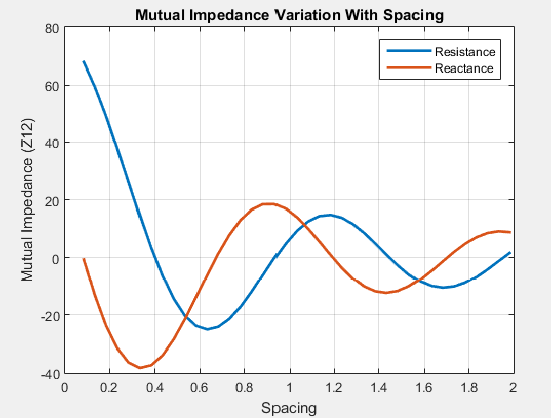The plot shows that as the spacing between elements increases, the mutual impedance, and hence mutual coupling decreases.

### Coupling Matrix

A coupling matrix is used to characterize the mutual coupling between the antenna elements at the port level. This matrix is calculated using S-parameters or Z-parameters and is used to decouple the array.

#### S-Parameter Matrix

To calculate the coupling matrix, you can use the S-parameter matrix. You calculate each column of the S-parameter matrix by feeding the antenna in that column by `1V`. Consider an element array arranged in a 2x2 grid. Visualize the grid and the element numbers using the `layout`.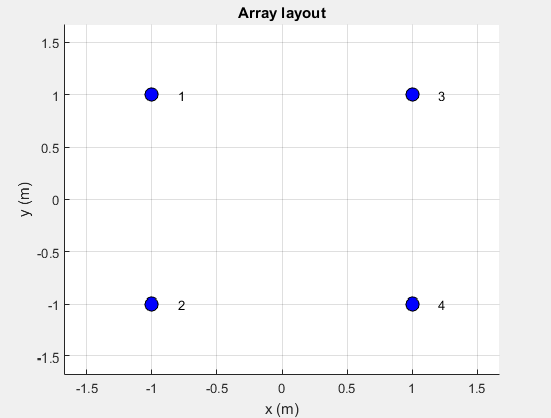There are four ports in this array. The corresponding S-parameter matrix would be of size 4 x 4:

`$S=\left(\begin{array}{cccc}{s}_{11}& {s}_{12}& {s}_{13}& {s}_{14}\\ {s}_{21}& {s}_{22}& {s}_{23}& {s}_{24}\\ {s}_{31}& {s}_{32}& {s}_{33}& {s}_{34}\\ {s}_{41}& {s}_{42}& {s}_{43}& {s}_{44}\end{array}\right)$`

During this measurement, terminate rest of the antenna elements using reference resistance of 50 ohms. The termination resistance is internally set to a default of 50 ohms and can be omitted during the calculation. If the termination is different, specify the resistance value when using this function. The diagonal terms in the matrix shown, represent the self-interaction which is also commonly referred to as the reflection coefficient. The off-diagonal terms capture the mutual coupling between the ports of the antenna.

Use `sparameters` to calculate the S-parameter coupling matrix of an array in Antenna Toolbox™.

### Array Factor and Pattern Multiplication

The basis of the array theory is the pattern multiplication theorem. This theorem states that the combined pattern of N identical array elements is expressed as the element pattern times the array factor.

The array factor is calculated using the formula:

`$AF=\sum _{i=0}^{N}V\left(i\right)·{e}^{\left(k\mathrm{sin}\theta \mathrm{cos}\phi ·x\left(i\right)+k\mathrm{sin}\phi ·y\left(i\right)+k\mathrm{cos}\theta ·z\left(i\right)\right)}$`

where:

• N is the number of elements in the array.

• V is the applied voltage (amplitude and phase) at each element in the array.

• k is the wave number.

• theta and phi are the elevation and azimuth angles.

• x, y, and z are the Cartesian coordinates of the feed locations for every antenna element of the array.

Once the array factor is calculated using the above equation, you can calculate the beam pattern of the array as the product of the array factor and the beam pattern of the individual antenna element of the array.

Array pattern = AF* individual antenna element pattern

The analysis assumes that the array elements are uncoupled. This means that the current in one element does not excite currents in the other elements or there is no mutual coupling between different elements of the array. This is the most serious limitation of the pattern multiplication theorem, restricting its use to arrays with large element spacing.

For example:

Calculate the array pattern of the rectangular array of dipoles in the x-y plane with a spacing of half-lambda

```fc = 1e9; lambda = physconst('lightspeed')/fc; az = -180:0.1:180; el = -90:0.1:90; %% Element d = design(dipole,1e9); d.Tilt = 90; d.TiltAxis = [0 1 0]; %% Array r = rectangularArray; r.Element = d; r.RowSpacing = lambda/2; r.ColumnSpacing = lambda/2; figure; show(r) ; figure; patternMultiply(r, fc, az, el); ```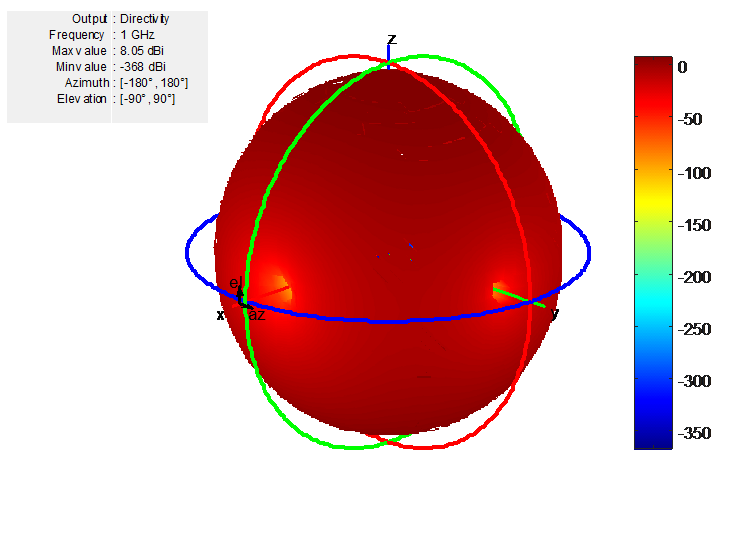Increase the size of the rectangular array to 200k elements. Below is the time taken for the computation and the calculated directivity.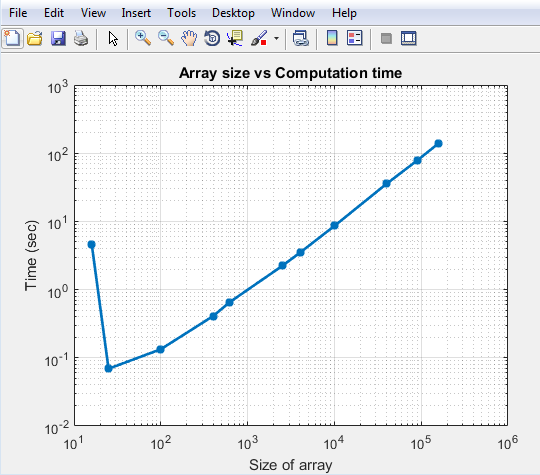You see that the time taken to solve a 100k array is less than 100 seconds. The initial bump in time for the small array is because you perform EM analysis to compute the pattern of the individual dipole element in the array. Once this analysis is done the results are cached and the successive calls do not perform any EM analysis. As a result the increase in time is fairly linear. This is the biggest advantage of using pattern multiplication. It lets you solve large arrays quickly and with limited memory requirements.

### Isolated Element Pattern

Patterns of individual elements in small arrays vary significantly. Therefore you cannot use pattern multiplication for complete array pattern because isolated element pattern assumes that all elements have the same pattern.

You can calculate the complete array pattern of small arrays by plotting the pattern all the elements separately in a small array. To obtain this pattern, each element is individually excited and the rest of the array elements terminated using reference impedance. The plot shows the radiation pattern of individual elements of 4--element array.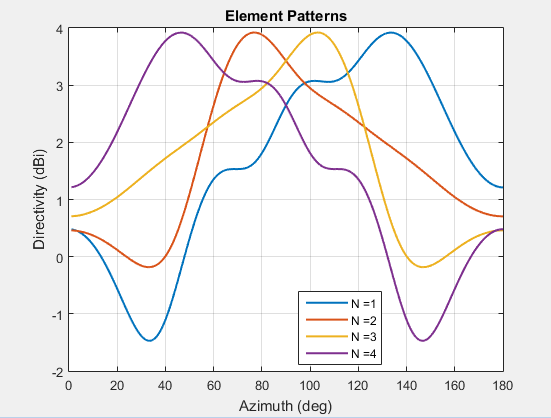### Embedded Element Pattern

The embedded element pattern is the pattern of a single element embedded in a finite array, calculated by driving a specific (typically the central) element in the array. The rest of the array elements are terminated using reference impedance. This method is useful for large array because the effect of mutual coupling on the individual element is captured. It is important to note that the edge effects can be ignored since the size of the array is assumed to be very large. It is common to use the center antenna element for this calculation. Due, to the size of the array the radiation patterns of the elements in the array can be approximated with the embedded element pattern instead of the isolated element pattern. Finally, pattern multiplication is used to calculate the complete array pattern.The figure shows the embedded element pattern of a center element in a `11x11` array. You can also calculate the embedded element pattern as a magnitude of electric field.

Isolated element pattern is not recommended for large arrays as this method does not account for the coupling effects of elements around it.

#### Scan Blindness

In large arrays, it is possible that the array directivity reduces drastically at certain scan angles. At these scan angles, referred to as the blind angles, the array does not radiate the power supplied at its input terminals . The scan blindness can occur while using these common mechanisms:

• Surface wave excitation

• Grating lobe excitation

To detect scan blindness in large finite arrays, study the embedded element pattern. In infinite array analysis, this pattern is known as the array element pattern.

 Stutzman, W.L. Thiele, G.A. Antenna Theory and Design, 3rd Edition. New York: Wiley, 2013, p. 307.